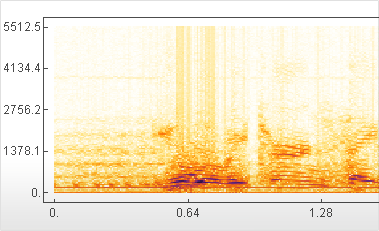# Built-in Signal Processing

Mathematica 9 introduces a new generation of signal processing and analysis, fully integrated with Mathematica's comprehensive continuous and discrete calculus and algebraic capabilities. New features include analog and digital filter design, and signal filtering and processing that can be applied to audio, image, and other data, as well as new signal visualization functions and discrete transforms.• Modern extensible platform for signal processing. »
• Signals, audio objects, images, and multidimensional data can be processed using hundreds of built-in Mathematica functions.
• Highly optimized and memory-efficient convolution-based filtering on signals and images. »
• Recursive filters can efficiently be applied to multidimensional data and images. »
• Efficient implementations of standard analog and digital filter design algorithms.
• Algebraic top-level representation of filters. »
• New functions for visual analysis of signals. »
• Integrated with Mathematica's comprehensive system for continuous and discrete calculus.
• A complete set of optimized algebraic continuous transforms. »
• A complete set of optimized algebraic discrete transforms. »
• New implementations of list-oriented discrete transforms. »
• New and optimized implementations of transform matrices. »
• A complete set of built-in window functions that can be used in FIR filter design, as well as processing and visualization of signals. »
• Use Mathematica's algebraic capabilities to perform higher- or exact-precision computations.
• Integrated with Mathematica's control systems capabilities. »
• Use Wolfram SystemModeler to simulate and verify analog filter design. »

## Mathematica

Questions? Comments? Contact a Wolfram expert »